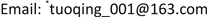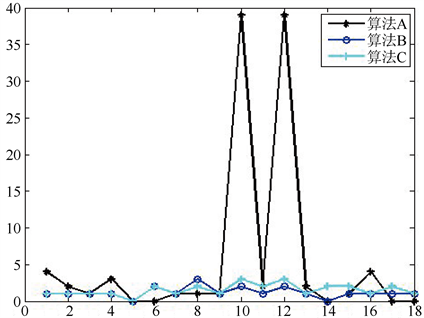﻿ 一类非奇异H-矩阵快速迭代判定新算法 A New Algorithm of Fast Iterative Criterion for Non-Singular H-Matrices

Vol. 08  No. 01 ( 2019 ), Article ID: 28567 , 9 pages
10.12677/AAM.2019.81011

A New Algorithm of Fast Iterative Criterion for Non-Singular H-Matrices

Xi Chen, Qing Tuo*

College of Mathematics and Statistics, Jishou University, Jishou HunanReceived: Dec. 29th, 2018; accepted: Jan. 15th, 2019; published: Jan. 22nd, 2019ABSTRACT

By improving the iterative matrix factors and convergence conditions, a new fast iterative criterion algorithm for non-singular H-matrices is obtained, and the convergence of the algorithm is theoretically explained. Finally, the results of Matlab numerical simulation experiments show that the proposed algorithm has faster convergence and better stability.

Keywords:Non-Singular H-Matrices, Iteration Matrices, Iterative Algorithms1. 引言

H-矩阵在计算数学，数学物理，控制论，统计学，弹性力学等众多领域中有着广泛应用。由于H-矩阵广泛的应用范围，其判定方法一直是人们关注的热点问题。因此，判别一个矩阵是否为H-矩阵及讨论其性质如何具有重大意义。由于近年来计算机的发展，国内外许多学者提出不少关于H-矩阵的迭代判定算法  -  。文献  以细化的思想通过对方阵行下标集及矩阵行和不同的递进式划分，得到了非奇异H-矩阵的若干判定定理和相应的迭代判定算法，增大对H-矩阵判定的范围，改进了文献   中的结果。本文主要基于文  思路，针对文献  的判定结果做出改进得到一类迭代判定新算法，同时改进了文献  的判定定理范围和文献  的部分算法结果，最后用数值仿真结果说明了新算法判定的稳定性和高效性。

${N}_{1}\left({A}^{\left(m\right)}\right)=\left\{i\in N:0<|{a}_{ii}^{\left(m\right)}|\le {\Lambda }_{i}\left({A}^{\left(m\right)}\right)\right\}$ , ${N}_{2}\left({A}^{\left(m\right)}\right)=\left\{i\in N:|{a}_{ii}^{\left(m\right)}|>{\Lambda }_{i}\left({A}^{\left(m\right)}\right)\right\}$ ,

${\Lambda }_{i}^{\left(1\right)}\left({A}^{\left(m\right)}\right)=\underset{t\in {N}_{1}\left({A}^{\left(m\right)}\right),t\ne i}{\sum }|{a}_{it}^{\left(m\right)}|+\underset{t\in {N}_{2}\left({A}^{\left(m\right)}\right)}{\sum }|{a}_{it}^{\left(m\right)}|\frac{{\Lambda }_{t}\left({A}^{\left(m\right)}\right)}{|{a}_{tt}^{\left(m\right)}|},\text{\hspace{0.17em}}\text{\hspace{0.17em}}\forall i\in {N}_{1}\left({A}^{\left(m\right)}\right)$ ,

${N}_{1}^{\left(1\right)}\left({A}^{\left(m\right)}\right)=\left\{i\in N:0<|{a}_{ii}^{\left(m\right)}|\le {\Lambda }_{i}^{\left(1\right)}\left({A}^{\left(m\right)}\right)\right\}$ , ${N}_{2}^{\left(1\right)}\left({A}^{\left(m\right)}\right)=\left\{i\in N:{\Lambda }_{i}\left({A}^{\left(m\right)}\right)\ge |{a}_{ii}^{\left(m\right)}|>{\Lambda }_{i}^{\left(1\right)}\left({A}^{\left(m\right)}\right)\right\}$ , ${\alpha }_{i}^{\left(m\right)}=\underset{t\in {N}_{1}\left({A}^{\left(m-1\right)}\right),t\ne i}{\sum }|{a}_{it}^{\left(m\right)}|$ , ${\beta }_{i}^{\left(m\right)}=\underset{t\in {N}_{2}\left({A}^{\left(m-1\right)}\right),t\ne i}{\sum }|{a}_{it}^{\left(m\right)}|,\text{\hspace{0.17em}}\text{\hspace{0.17em}}i\in N$

${d}_{i}=\left\{\begin{array}{l}1\text{\hspace{0.17em}}\text{\hspace{0.17em}}\text{\hspace{0.17em}}\text{\hspace{0.17em}}\text{\hspace{0.17em}}\text{\hspace{0.17em}}\text{\hspace{0.17em}}\text{\hspace{0.17em}}\text{\hspace{0.17em}}\text{\hspace{0.17em}}\text{\hspace{0.17em}}\text{\hspace{0.17em}}\text{\hspace{0.17em}}\text{\hspace{0.17em}}\text{\hspace{0.17em}}\text{\hspace{0.17em}}\text{\hspace{0.17em}}\text{\hspace{0.17em}}\text{\hspace{0.17em}}i\in {N}_{1}^{\left(1\right)}\left({A}^{\left(m-1\right)}\right)\\ \frac{{\Lambda }_{i}^{\left(1\right)}\left({A}^{\left(m\right)}\right)}{|{a}_{ii}^{\left(m\right)}|}\text{\hspace{0.17em}}\text{\hspace{0.17em}}\text{\hspace{0.17em}}\text{\hspace{0.17em}}\text{\hspace{0.17em}}\text{\hspace{0.17em}}i\in {N}_{2}^{\left(1\right)}\left({A}^{\left(m-1\right)}\right)\\ \frac{{\Lambda }_{i}\left({A}^{\left(m\right)}\right)}{|{a}_{ii}^{\left(m\right)}|}\text{\hspace{0.17em}}\text{\hspace{0.17em}}\text{\hspace{0.17em}}\text{\hspace{0.17em}}\text{\hspace{0.17em}}\text{ }\text{\hspace{0.17em}}\text{\hspace{0.17em}}\text{ }i\in {N}_{2}\left({A}^{\left(m-1\right)}\right)\end{array}$

2. 主要结果

${M}_{n}\left(C\right)\left({M}_{n}\left(R\right)\right)$ 为n阶复(实)矩阵的集合。 $A=\left({a}_{ij}\right)\in {M}_{n}\left(C\right)$ ，记

$N=\left\{1,2,\cdots ,n\right\}$ , ${\Lambda }_{i}\left(A\right)=\underset{j\ne i}{\sum }|{a}_{ij}|,\text{\hspace{0.17em}}\forall i\in N$ ,

${N}_{1}=\left\{i\in N|0<|{a}_{ii}|={\Lambda }_{i}\left(A\right)\right\}$ , ${N}_{2}=\left\{i\in N|0<|{a}_{ii}|<{\Lambda }_{i}\left(A\right)\right\}$ ,

${N}_{3}=\left\{i\in N||{a}_{ii}|>{\Lambda }_{i}\left(A\right)\right\}$ , $N={N}_{1}\oplus {N}_{2}\oplus {N}_{3}$

$r=\underset{i\in {N}_{3}}{\mathrm{max}}\left\{\frac{\underset{t\in {N}_{2}}{\sum }|{a}_{it}|+\underset{t\in {N}_{1}}{\sum }|{a}_{it}|}{|{a}_{ii}|-\underset{t\in {N}_{3},t\ne i}{\sum }|{a}_{it}|}\right\}$ , ${r}_{1}=\underset{i\in {N}_{3}}{\mathrm{max}}\left\{\frac{\underset{t\in {N}_{2}}{\sum }|{a}_{it}|+\underset{t\in {N}_{1}}{\sum }|{a}_{it}|}{|{a}_{ii}|-\underset{t\in {N}_{3},t\ne i}{\sum }|{a}_{it}|\frac{{P}_{t,r}\left(A\right)}{|{a}_{tt}|}}\right\}$ ,

${P}_{i,r}\left(A\right)=\underset{t\in {N}_{1}}{\sum }|{a}_{it}|+\underset{t\in {N}_{2}}{\sum }|{a}_{it}|+r\underset{t\in {N}_{3},t\ne i}{\sum }|{a}_{it}|\text{\hspace{0.17em}}\left(i\in {N}_{3}\right)$ ,

${P}_{i,{r}_{1}}\left(A\right)=\underset{t\in {N}_{1}}{\sum }|{a}_{it}|+\underset{t\in {N}_{2}}{\sum }|{a}_{it}|+{r}_{1}\underset{t\in {N}_{3},t\ne i}{\sum }|{a}_{it}|\frac{{P}_{t,r}}{|{a}_{tt}|}\text{\hspace{0.17em}}\left(i\in {N}_{3}\right)$ ,

${w}_{i}=\frac{{\Lambda }_{i}\left(A\right)}{{\Lambda }_{i}\left(A\right)+|{a}_{ii}|}\text{\hspace{0.17em}}\left(i\in {N}_{2}\right)$ , $\delta =\mathrm{max}\left\{{r}_{1},{w}_{i}\right\}$ , $h=\underset{i\in {N}_{3}}{\mathrm{max}}\left\{\frac{\delta \left(\underset{t\in {N}_{1}}{\sum }|{a}_{it}|\right)+\underset{t\in {N}_{2}}{\sum }|{a}_{it}|{w}_{t}}{{P}_{i,{r}_{1}}\left(A\right)-\underset{t\in {N}_{3},t\ne i}{\sum }|{a}_{it}|\frac{{P}_{t,{r}_{1}}\left(A\right)}{|{a}_{tt}|}}\right\}$ .

${S}_{i}\left(A\right)=|{a}_{it}|{w}_{i}-\delta \underset{t\in {N}_{1}}{\sum }|{a}_{it}|-\underset{t\in {N}_{2},t\ne i}{\sum }|{a}_{it}|{w}_{t}-h\underset{t\in {N}_{3}}{\sum }|{a}_{it}|\frac{{P}_{t,{r}_{1}}\left(A\right)}{|{a}_{tt}|}\text{\hspace{0.17em}}\left(i\in {N}_{2}\right)$ , ${R}_{i}=\frac{1}{\underset{t\in {N}_{3}}{\sum }|{a}_{it}|}{S}_{i}$ .

2.1. 无参数算法

$|{a}_{ii}^{\left(m\right)}|{w}_{i}^{\left(m\right)}>{\delta }^{\left(m\right)}\underset{t\in {N}_{1}\left({A}^{\left(m\right)}\right)}{\sum }|{a}_{it}^{\left(m\right)}|+\underset{t\in {N}_{2}\left({A}^{\left(m\right)}\right),t\ne i}{\sum }|{a}_{it}^{\left(m\right)}|{w}_{t}^{\left(m\right)}+{h}^{\left(m\right)}\underset{t\in {N}_{3}\left({A}^{\left(m\right)}\right)}{\sum }|{a}_{it}^{\left(m\right)}|\frac{{P}_{t,{r}_{1}}^{\left(m\right)}}{|{a}_{tt}^{\left(m\right)}|},\forall i\in {N}_{2}\left({A}^{\left(m\right)}\right)$ ,

${N}_{1}\left({A}^{\left(m\right)}\right)=\varphi$$i\in {N}_{1}\left({A}^{\left(m\right)}\right)\ne \varphi$ 时，存在 $t\in {N}_{2}\left({A}^{\left(m\right)}\right)\cup {N}_{3}\left({A}^{\left(m\right)}\right)$ ，使得 ${a}_{it}^{\left(m\right)}\ne 0$ ，则“A是非奇异H-矩阵”，停止；否则，

${d}_{i}^{\left(m\right)}=\left\{\begin{array}{l}{\delta }^{\left(m\right)}\text{\hspace{0.17em}}\text{\hspace{0.17em}}\text{\hspace{0.17em}}\text{\hspace{0.17em}}\text{\hspace{0.17em}}\text{\hspace{0.17em}}\text{\hspace{0.17em}}\text{\hspace{0.17em}}\text{\hspace{0.17em}}\text{\hspace{0.17em}}\text{\hspace{0.17em}}\text{ }\text{\hspace{0.17em}}i\in {N}_{1}\left({A}^{\left(m\right)}\right)\\ {w}_{i}^{\left(m\right)}\text{\hspace{0.17em}}\text{\hspace{0.17em}}\text{\hspace{0.17em}}\text{\hspace{0.17em}}\text{\hspace{0.17em}}\text{\hspace{0.17em}}\text{\hspace{0.17em}}\text{\hspace{0.17em}}\text{\hspace{0.17em}}\text{\hspace{0.17em}}\text{\hspace{0.17em}}\text{\hspace{0.17em}}i\in {N}_{2}\left({A}^{\left(m\right)}\right)\\ \frac{{h}^{\left(m\right)}{P}_{i,{r}_{1}}^{\left(m\right)}}{|{a}_{ii}^{\left(m\right)}|}\text{\hspace{0.17em}}\text{\hspace{0.17em}}\text{\hspace{0.17em}}\text{\hspace{0.17em}}\text{\hspace{0.17em}}\text{\hspace{0.17em}}\text{ }i\in {N}_{3}\left({A}^{\left(m\right)\right)}\end{array}$

$\frac{{\Lambda }_{i}\left({A}^{\left(m\right)}\right)}{|{a}_{ii}^{\left(m\right)}|}<\frac{{h}^{\left(m\right)}{P}_{i,{r}_{1}}^{\left(m\right)}}{|{a}_{ii}^{\left(m\right)}|}$ .

2.2. 带参数算法

$|{a}_{ii}^{\left(m\right)}|{w}_{i}^{\left(m\right)}>{\delta }^{\left(m\right)}\underset{t\in {N}_{1}\left({A}^{\left(m\right)}\right)}{\sum }|{a}_{it}^{\left(m\right)}|+\underset{t\in {N}_{2}\left({A}^{\left(m\right)}\right),t\ne i}{\sum }|{a}_{it}^{\left(m\right)}|{w}_{t}^{\left(m\right)}+{h}^{\left(m\right)}\underset{t\in {N}_{3}\left({A}^{\left(m\right)}\right)}{\sum }|{a}_{it}^{\left(m\right)}|\frac{{P}_{t,{r}_{1}}^{\left(m\right)}}{|{a}_{tt}^{\left(m\right)}|},\forall i\in {N}_{2}\left({A}^{\left(m\right)}\right)$ ,

${N}_{1}\left({A}^{\left(m\right)}\right)=\varphi$$i\in {N}_{1}\left({A}^{\left(m\right)}\right)\ne \varphi$ 时，存在 $t\in {N}_{2}\left({A}^{\left(m\right)}\right)\cup {N}_{3}\left({A}^{\left(m\right)}\right)$ ，使得 ${a}_{it}^{\left(m\right)}\ne 0$ ，则“A是非奇H-矩阵”，停止；否则，

${d}_{i}^{\left(m\right)}=\left\{\begin{array}{l}{\delta }^{\left(m\right)}\text{\hspace{0.17em}}\text{\hspace{0.17em}}\text{\hspace{0.17em}}\text{\hspace{0.17em}}\text{\hspace{0.17em}}\text{\hspace{0.17em}}\text{\hspace{0.17em}}\text{\hspace{0.17em}}\text{\hspace{0.17em}}\text{\hspace{0.17em}}\text{\hspace{0.17em}}\text{\hspace{0.17em}}\text{\hspace{0.17em}}\text{\hspace{0.17em}}\text{\hspace{0.17em}}\text{\hspace{0.17em}}\text{\hspace{0.17em}}\text{\hspace{0.17em}}\text{\hspace{0.17em}}\text{\hspace{0.17em}}\text{ }i\in {N}_{1}\left({A}^{\left(m\right)}\right)\\ {w}_{i}^{\left(m\right)}\text{\hspace{0.17em}}\text{\hspace{0.17em}}\text{\hspace{0.17em}}\text{\hspace{0.17em}}\text{\hspace{0.17em}}\text{\hspace{0.17em}}\text{\hspace{0.17em}}\text{\hspace{0.17em}}\text{\hspace{0.17em}}\text{\hspace{0.17em}}\text{\hspace{0.17em}}\text{\hspace{0.17em}}\text{\hspace{0.17em}}\text{\hspace{0.17em}}\text{\hspace{0.17em}}\text{\hspace{0.17em}}\text{\hspace{0.17em}}\text{\hspace{0.17em}}\text{\hspace{0.17em}}\text{\hspace{0.17em}}i\in {N}_{2}\left({A}^{\left(m\right)}\right)\\ \frac{{h}^{\left(m\right)}{P}_{i,{r}_{1}}^{\left(m\right)}}{|{a}_{ii}^{\left(m\right)}|}+{\epsilon }^{\left(m\right)}\text{\hspace{0.17em}}\text{\hspace{0.17em}}\text{\hspace{0.17em}}\text{\hspace{0.17em}}\text{\hspace{0.17em}}\text{\hspace{0.17em}}i\in {N}_{3}\left({A}^{\left(m\right)\right)}\end{array}$

${\epsilon }^{\left(m\right)}=\left\{\begin{array}{l}\frac{1}{q}\mathrm{min}{S}_{i}^{\left(m\right)}\text{\hspace{0.17em}}\text{\hspace{0.17em}}\text{\hspace{0.17em}}\text{\hspace{0.17em}}\text{\hspace{0.17em}}\text{\hspace{0.17em}}\text{\hspace{0.17em}}\exists i\in {N}_{2}\left({A}^{\left(m\right)}\right),\underset{t\in {N}_{3}}{\sum }|{a}_{it}^{\left(m\right)}|=0\\ \mathrm{min}{R}_{i}^{\left(m\right)}\text{\hspace{0.17em}}\text{\hspace{0.17em}}\text{\hspace{0.17em}}\text{\hspace{0.17em}}\text{\hspace{0.17em}}\text{\hspace{0.17em}}\text{\hspace{0.17em}}\text{\hspace{0.17em}}\text{\hspace{0.17em}}\forall i\in {N}_{2}\left({A}^{\left(m\right)}\right),\underset{t\in {N}_{3}}{\sum }|{a}_{it}^{\left(m\right)}|\ne 0\end{array}$

3. 算法收敛性分析

$A={A}^{\left(0\right)}={A}^{\left(1\right)}\ge \cdots \ge {A}^{\left(m\right)}\ge \cdots \ge 0$ ,

$\underset{m\to \infty }{\mathrm{lim}}{A}^{\left(m\right)}=B\ge 0$ ,

$\underset{m\to \infty }{\mathrm{lim}}{N}_{3}\left({A}^{\left(m\right)}\right)={N}_{3}\left(B\right)=\varphi$ .

$|{a}_{ii}^{\left(m\right)}|\left(1-{\delta }^{\left(m\right)}\right)>{\epsilon }_{1}$ , $|{a}_{ii}^{\left(m\right)}|\left(1-{w}_{i}^{\left(m\right)}\right)>{\epsilon }_{2}$ , $|{a}_{ii}^{\left(m\right)}|\left(1-\frac{{h}^{\left(m\right)}{P}_{i,{r}_{1}}^{\left(m\right)}}{|{a}_{ii}^{\left(m\right)}|}\right)>{\epsilon }_{3}$ , $m=1,2,3,\cdots$

${\epsilon }_{0}=\mathrm{min}\left\{{\epsilon }_{1},{\epsilon }_{2},{\epsilon }_{3}\right\}$

$0<{a}_{ii}^{\left(m+1\right)}={a}_{ii}^{\left(m\right)}{\delta }^{\left(m\right)}<{a}_{ii}^{\left(m\right)}-{\epsilon }_{1}<{a}_{ii}^{\left(m\right)}-{\epsilon }_{0}$ ,

$0<{a}_{ii}^{\left(m+1\right)}={a}_{ii}^{\left(m\right)}{w}_{i}^{\left(m\right)}<{a}_{ii}^{\left(m\right)}-{\epsilon }_{2}<{a}_{ii}^{\left(m\right)}-{\epsilon }_{0}$ ,

$0<{a}_{ii}^{\left(m+1\right)}={a}_{ii}^{\left(m\right)}\frac{{h}^{\left(m\right)}{P}_{i,{r}_{1}}^{\left(m\right)}}{|{a}_{ii}^{\left(m\right)}|}<{a}_{ii}^{\left(m\right)}-{\epsilon }_{3}<{a}_{ii}^{\left(m\right)}-{\epsilon }_{0}$ .

${a}_{ii}^{\left(0\right)}={a}_{ii}^{\left(1\right)}>{a}_{ii}^{\left(2\right)}+{\epsilon }_{0}>\cdots >{a}_{ii}^{\left(m\right)}+\left(m-1\right){\epsilon }_{0}$ ,

$m\to \infty$ ，则 ${a}_{ii}\to \infty$ ，产生矛盾。故

$\underset{m\to \infty }{\mathrm{lim}}{N}_{3}\left({A}^{\left(m\right)}\right)={N}_{3}\left(B\right)=\varphi$ .

4. 数值仿真Figure 1. Curve: system result of iterative AlgorithmTable 4. Data of Algorithmic comprehensive performance

5. 结论

A New Algorithm of Fast Iterative Criterion for Non-Singular H-Matrices[J]. 应用数学进展, 2019, 08(01): 96-104. https://doi.org/10.12677/AAM.2019.81011

1. 1. Ojiro, K., Niki, H. and Usui, M. (2003) A New Criterion for the H-Matrix Property. Journal of Computational and Ap-plied Mathematics, 150, 293-302.
https://doi.org/10.1016/S0377-0427(02)00666-0

2. 2. Kohno, T., et al. (2000) An Iterative Test for H-Matrices. Journal of Computational and Applied Mathematics, 115, 349-355.
https://doi.org/10.1016/S0377-0427(99)00303-9

3. 3. Liu, J.Z. and He, A.Q. (2006) A New Algorithmic Charac-terization of H-Matrices. Applied Mathematics and Computation, 183, 603-609.
https://doi.org/10.1016/j.amc.2006.05.100

4. 4. Liu, J.Z. and He, A.Q. (2007) An Iterleaved Iterative Criterion for H-Matrices. Applied Mathematics and Computation, 186, 727-734.
https://doi.org/10.1016/j.amc.2006.08.031

5. 5. 周伟伟, 徐仲, 等. 非奇H-矩阵细分迭代判定准则[J]. 数值计算与计算机应用, 2011, 32(4): 293-300.

6. 6. 张骁, 陆全, 等. 非奇H-矩阵的一组迭代判别法[J]. 2015, 36(1): 59-68.

7. 7. 丁碧文, 刘建州. H-矩阵的判别法及其迭代算法[J]. 应用数学学报, 2013, 36(5): 935-948.

8. 8. Gao, Y.-M. and Wang, X.-H. (1992) Criteria for Generalized Diagonally Dominant Matrices and M-Matrices. Linear Algebra and its Applications, 169, 257-268.
https://doi.org/10.1016/0024-3795(92)90182-A

9. 9. 沈光星. 非奇异H阵的新判据[J]. 工程数学学报, 1998(4): 23-29.

10. 10. 庹清, 朱砾, 刘建州. 一类非奇异H-矩阵判定的新条件[J]. 计算数学, 2008(2): 177-182.

NOTES

*通讯作者。